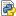# calibration.pycalibration.py — Python Source, 2 KB (2647 bytes)

## File contents

```#!/usr/bin/env python3
import scipy
import numpy as np

__all__ = ["calibrate"]

DEFAULT_PT100_COEFFICIENTS = (
-264.8895,
234.1986,
-2.480645,
-0.1422663,
)

def polynom(x, coeffs):
return coeffs * x**3 + coeffs * x**2 + coeffs * x + coeffs

def polynom_with_offset(x, coeffs, offset):
return polynom(x, coeffs) - offset

def format_command_parameter(value):
"""Forces numbers to fit into 9 characters, so OsTech commands won't get too long."""
result = ""
for i in range(9, 0, -1):
result = "%.*g" % (i, value)
if len(result) <= 9:
break
return result

def get_commands(coeffs, sensor_number=None):
"""Generates OsTech Sensor coefficient commands."""
sensor_number_string = str(sensor_number) if sensor_number else "[SensorNumber]"
coeffs_formatted = [format_command_parameter(c) for c in coeffs]
commands = [
f"{sensor_number_string}SSC{i}{c}" for i, c in enumerate(coeffs_formatted)
]
return commands

def calibrate(
temperatures,
sensor_number=None,
coeffs=DEFAULT_PT100_COEFFICIENTS,
):
"""Calculate new sensor coefficients from measured temperature pairs and old coefficients.

:param temperatures: list of temperature pairs [(OsTech device temperature, externally measured temperature)]
:param sensor_number: number of the temperature sensor (1…7)
:param coeffs: old sensor coefficients (c0…c3), can be omitted if default coefficients are used
:return: list of new sensor coefficients (c0…c3), commands to set the new coefficients
"""
temperatures = np.array(temperatures)
temp_difference = temperatures[:, 0] - temperatures[:, 1]
voltages = np.array(
[
scipy.optimize.root(
polynom_with_offset, np.array(), args=(coeffs, temp)
).x
for temp, _ in temperatures
]
)

coeffs_difference = np.array((np.polyfit(voltages, temp_difference, 3))[::-1])
coeffs_new = [float(c) for c in coeffs - coeffs_difference]

result = (
coeffs_new,
get_commands(coeffs_new, sensor_number),
)
return result

# Example
if __name__ == "__main__":
temperatures = [
# (temperature measured by OsTech device, externally measured temperature)
(-5.0, -5.8),
(35.0, 35.5),
(75.0, 77.6),
]

coeffs_new, commands = calibrate(temperatures, sensor_number=3)
coeffs_lines = "\n".join([str(x) for x in coeffs_new])
print(f"Coefficients:\n{coeffs_lines}")
command_lines = "\n".join(commands)
print(f"Commands:\n{command_lines}")
```